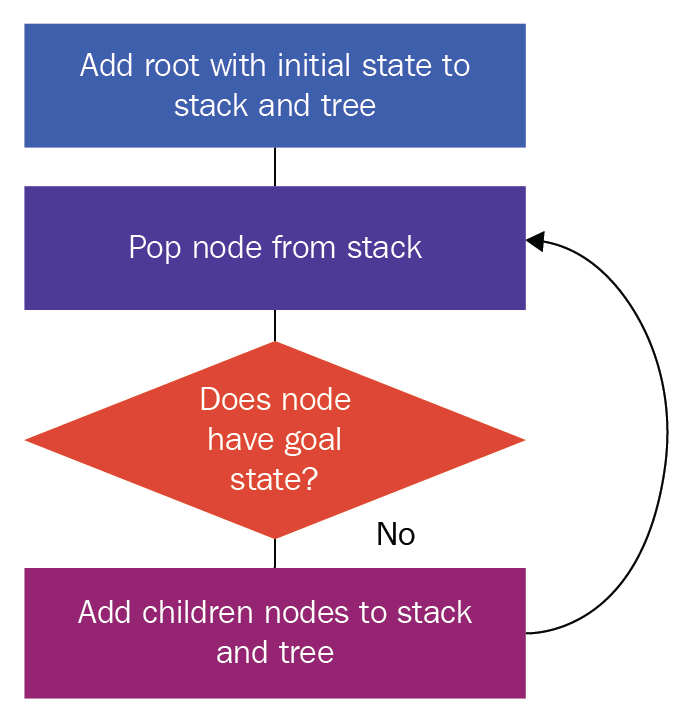# The DFS algorithm

Now that you understand the basic concepts of searching, we'll look at how DFS works by using the three basic ingredients of search algorithms—the initial state, the successor function, and the goal function. We will use the stack data structure.

Let's first represent the DFS algorithm in the form of a flowchart, to offer a better understanding:Figure 27

The steps in the preceding flowchart are as follows:

1. We create a root node using the initial state, and we add this to our stack and tree
2. We pop a node from the stack
3. We check whether it has the goal state; if it has the goal state, we stop our search here